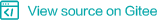## Overview¶

MindSpore helps you load common datasets, datasets of specific data formats, or custom datasets. Before loading a dataset, you need to import the required library `mindspore.dataset`.

```import mindspore.dataset as ds
```

MindSpore can load common standard datasets. The following table lists the supported datasets:

Dataset Description
ImageNet An image database organized based on the WordNet hierarchical structure. Each node in the hierarchical structure is represented by hundreds of images.
MNIST A large database of handwritten digit images, which is usually used to train various image processing systems.
CIFAR-10 A collection of images that are commonly used to train machine learning and computer vision algorithms. The CIFAR-10 dataset contains 60,000 32x32 color images in 10 different classes.
CIFAR-100 The dataset is similar to CIFAR-10. The difference is that this dataset has 100 classes, and each class contains 600 images, including 500 training images and 100 test images.
PASCAL-VOC The data content is diversified and can be used to train computer vision models (such as classification, positioning, detection, segmentation, and action recognition).
CelebA CelebA face dataset contains tens of thousands of face images of celebrities with 40 attribute annotations, which are usually used for face-related training tasks.

The procedure for loading common datasets is as follows. The following describes how to create the `CIFAR-10` object to load supported datasets.

1. Download and decompress the CIFAR-10 Dataset. The dataset in binary format (CIFAR-10 binary version) is used.

2. Configure the dataset directory and define the dataset instance to be loaded.

```DATA_DIR = "cifar10_dataset_dir/"

cifar10_dataset = ds.Cifar10Dataset(DATA_DIR)
```
3. Create an iterator and read data through the iterator.

```for data in cifar10_dataset.create_dict_iterator():
# In CIFAR-10 dataset, each dictionary of data has keys "image" and "label".
print(data["image"])
print(data["label"])
```

### MindSpore Data Format¶

MindSpore supports reading of datasets stored in MindSpore data format, that is, `MindRecord` which has better performance and features.

For details about how to convert datasets to the MindSpore data format, see the Converting the Dataset to MindSpore Data Format.

To read a dataset using the `MindDataset` object, perform the following steps:

1. Create `MindDataset` for reading data.

```CV_FILE_NAME = os.path.join(MODULE_PATH, "./imagenet.mindrecord")
data_set = ds.MindDataset(dataset_file=CV_FILE_NAME)
```

In the preceding information:
`dataset_file`: specifies the MindRecord file, including the path and file name.

2. Create a dictionary iterator and read data records through the iterator.

```num_iter = 0
for data in data_set.create_dict_iterator():
print(data["label"])
num_iter += 1
```

### `Manifest` Data Format¶

`Manifest` is a data format file supported by Huawei ModelArts. For details, see https://support.huaweicloud.com/engineers-modelarts/modelarts_23_0009.html.

MindSpore provides dataset classes for datasets in Manifest format. Run the following commands to configure the dataset directory and define the dataset instance to be loaded:

```DATA_DIR = "manifest_dataset_path"

manifest_dataset = ds.ManifestDataset(DATA_DIR)
```

Currently, ManifestDataset supports only datasets of images and labels. The default column names are “image” and “label”.

### `TFRecord` Data Format¶

MindSpore can also read datasets in the `TFRecord` data format through the `TFRecordDataset` object.

1. Input the dataset path or the .tfrecord file list to create the `TFRecordDataset`.

```DATA_DIR = ["tfrecord_dataset_path/train-0000-of-0001.tfrecord"]

dataset = ds.TFRecordDataset(DATA_DIR)
```
2. Create schema files or schema classes to set the dataset format and features.

The following is an example of the schema file:

```{
"datasetType": "TF",
"numRows": 3,
"columns": {
"image": {
"type": "uint8",
"rank": 1
},
"label" : {
"type": "int64",
"rank": 1
}
}
}
```

In the preceding information:
`datasetType`: data format. TF indicates the TFRecord data format.
`columns`: column information field, which is defined based on the actual column names of the dataset. In the preceding schema file example, the dataset columns are image and label.
`numRows`: row information field, which controls the maximum number of rows for loading data. If the number of defined rows is greater than the actual number of rows, the actual number of rows prevails during loading.

When creating the TFRecordDataset, input the schema file path. An example is as follows:

```DATA_DIR = ["tfrecord_dataset_path/train-0000-of-0001.tfrecord"]
SCHEMA_DIR = "dataset_schema_path/schema.json"

dataset = ds.TFRecordDataset(DATA_DIR, schema=SCHEMA_DIR)
```

An example of creating a schema class is as follows:

```import mindspore.common.dtype as mstype
schema = ds.Schema()
schema.add_column('image', de_type=mstype.uint8)  # Binary data usually use uint8 here.

dataset = ds.TFRecordDataset(DATA_DIR, schema=schema)
```
3. Create a dictionary iterator and read data through the iterator.

```for data in dataset.create_dict_iterator():
# The dictionary of data has keys "image" and "label" which are consistent with columns names in its schema.
print(data["image"])
print(data["label"])
```

You can load a custom dataset using the `GeneratorDataset` object.

1. Define a function (for example, `Generator1D`) to generate a dataset.

The custom generation function returns the objects that can be called. Each time, tuples of `numpy array` are returned as a row of data.

An example of a custom function is as follows:

```import numpy as np  # Import numpy lib.
def Generator1D():
for i in range(64):
yield (np.array([i]),)  # Notice, tuple of only one element needs following a comma at the end.
```
2. Transfer `Generator1D` to `GeneratorDataset` to create a dataset and set `column` to data.

```dataset = ds.GeneratorDataset(Generator1D, ["data"])
```
3. After creating a dataset, create an iterator for the dataset to obtain the corresponding data. Iterator creation methods are as follows:

• Create an iterator whose return value is of the sequence type.

```for data in dataset.create_tuple_iterator():  # each data is a sequence
print(data)
```
• Create an iterator whose return value is of the dictionary type.

```for data in dataset.create_dict_iterator():  # each data is a dictionary
print(data["data"])
```